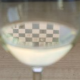# Online Cycle Detection in Directed Graphs

A short while ago I came across a quite interesting problem. Design a datastructure (and algorithms) to maintain a Directed Acyclic Graph.

There has to be only one operation: adding a link between two given nodes. This operation must be able to detect and deny any new link that would cause a cycle. For simplicity, nodes are identified by sequential ids starting with 0.

An example:

addLink 0, 1 -> True addLink 1, 2 -> True addLink 2, 0 -> False # Fails because it would create a cycle

addLink 0, 1 -> True addLink 1, 2 -> True addLink 0, 2 -> True # This isn't a real cycle, so it's perfectly fine

It’s rather trivial to create a $$\mathcal{O}\left(n+\ell\right)$$ (where $$n$$ is the number of nodes and $$\ell$$ the number of links). I conjecture there exists a $$\mathcal{O}\left(\log n\right)$$ algorithm.

## One thought on “Online Cycle Detection in Directed Graphs”

1.Ian Zimmerman says:

I think you got this from the same place as I did, and this was one more reason to not apply 🙂 Anyway, this seems to be still an active research topic; try to google for something like ‘digraph incremental topological sort’. Note that it only makes sense to talk about complexity _per iteration_. There are indeed a few algorithms more efficient than the naive O (n+l) per iteration.